# the seven living generations in america

## American generations

A quick look at the composition of American generations. Per Pew Research definitions & US Census data. In the process, a static perspective on the changing demographics of America.

library(tidyverse)
gen <- c('Post-Z', 'Gen Z', 'Millennial',
'Gen X', 'Boomers', 'Silent',
'Greatest')

range <- c('> 2012', '1997-2012', '1981-1996',
'1965-1980', '1946-1964', '1928-1945',
'< 1927')

gen_desc <- data.frame(rank = 7:1,
gen = gen,
range = range,
stringsAsFactors = FALSE) %>%
arrange(rank)

A summary:

Four of America’s seven living generations are more or less “complete,” and only getting smaller (albeit at different rates): Greatest, Silent, Boomers, and Gen X. The generation comprised of Millenials is complete as well, in that it has been delineated chronologically; however, the group likely continues to grow via immigration.

While Gen Z has been tentatively stamped chronologically by the folks at Pew Research, only the very eldest in the group have just entered the work force. So lot’s can happen still. And although we include them here, the Post-Z generation is mostly but a thought; half of the group has yet to be born.

## Monthly US population estimates

Monthly Postcensal Resident Population plus Armed Forces Overseas, December 2018. Made available by the US Census here. The census has transitioned to a new online interface, and (seemingly) many data sets have been discontinued. Hence, the data set utilized here is slightly dated.

pops <- read.csv (
url('https://www2.census.gov/programs-surveys/popest/datasets/2010-2018/national/asrh/nc-est2018-alldata-p-File18.csv')) %>%
filter(MONTH == '12' & YEAR == '2018') %>%
gather(key = 'race', value = 'pop', -UNIVERSE:-AGE)

A more detailed description of the population estimates can be found here. Note: Race categories reflect non-Hispanic populations.

race <- c('NHWA', 'NHBA', 'NHIA',
'NHAA', 'NHNA', 'NHTOM', 'H')

race1 <- c('White Alone',
'Black Alone',
'American Indian Alone',
'Asian Alone',
'Native Hawaiian Alone',
'Two or More Races',
'Hispanic')

labels <- data.frame(race = race,
race1=race1,
stringsAsFactors = FALSE)

search <- paste(paste0('^',race, '_'), collapse =  '|')

The following table details a random sample of the data set – with Pew Research defined generations & estimated year-of-birth.

gen_pops <- pops %>%
filter(grepl(search, race)) %>%
mutate(race = gsub('_.*$', '', race)) %>% group_by(AGE, race) %>% summarise(pop = sum(pop))%>% left_join(labels) %>% filter(AGE != '999') %>% mutate(yob = 2019 - AGE) %>% ## mutate (gen = case_when ( yob < 2013 & yob > 1996 ~ 'Gen Z', yob < 1997 & yob > 1980 ~ 'Millennial', yob < 1981 & yob > 1964 ~ 'Gen X', yob < 1965 & yob > 1945 ~ 'Boomers', yob < 1946 & yob > 1927 ~ 'Silent', yob < 1928 ~ 'Greatest', yob > 2012 ~ 'Post-Z')) %>% left_join(gen_desc) %>% ungroup() %>% select(gen, rank, range, race, race1, yob, AGE, pop) set.seed(999) gen_pops %>% sample_n(7) %>% select(gen, range, race1:pop) %>% DT::datatable(rownames = FALSE, options = list(dom = 't', scrollX = TRUE)) ## Composition of American generations ### Population by generation The figure below summarizes the US population by generation. These numbers will vary some depending on the data source. Millenials constitute the plurality of Americans, more recently overtaking a Boomer generation on the wane. gen_pops %>% group_by(gen, rank) %>% summarize(pop = sum(pop)) %>% mutate(lab = round(pop/1000000, 1)) %>% ggplot(aes(x = reorder(gen, rank), y = pop, fill = gen)) + geom_col(show.legend = FALSE, alpha = 0.75) + geom_text(aes(label = lab), size = 3.5)+ theme(axis.text.x=element_blank(), axis.ticks.x=element_blank())+ xlab('') + ylab('') + coord_flip()+ ggthemes::scale_fill_stata() + theme_minimal() + labs(title = 'Population by American generation', caption = 'SOURCE: US Census, Monthly Postcensal Resident Population plus Armed Forces Overseas, December 2018.')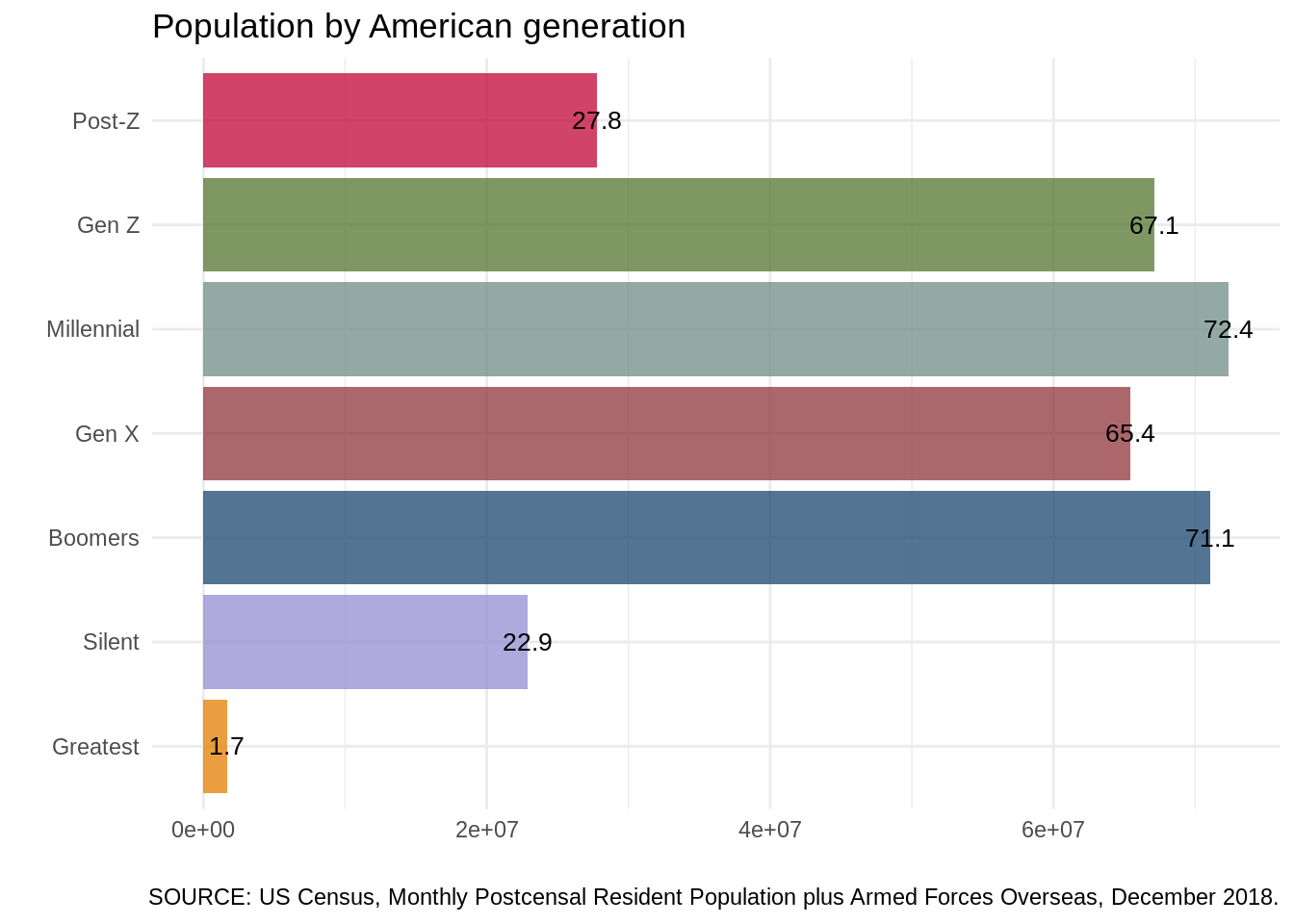### Population by single year of age & generation gg <- gen_pops %>% group_by(yob, AGE, gen) %>% summarize(tot = sum(pop)) %>% group_by(gen) %>% mutate(tot = max(tot)) %>% #For labels below. filter(yob %in% c('1919', '1928', '1946', '1965', '1981', '1997', '2013')) The figure below illustrates the US population by single year of age, ranging from the population aged less than a year to the population over 100 (as of December 2018). Generation membership per single year of age is specified by color. gen_pops %>% ggplot(aes(x = AGE, y = pop, fill = gen)) + geom_vline(xintercept = gg$AGE,
linetype =2,
color = 'gray',
size = .25)+

geom_col(show.legend = FALSE,
alpha = 0.85,
width = .7)   +
annotate(geom="text",
x = gg$AGE - 4.5, y = gg$tot + 70000,
label = gg$gen, size = 3.25) + xlab('Age')+ ylab('') + theme_minimal() + theme(legend.position="bottom", legend.title = element_blank(), panel.grid.major.x=element_blank(), panel.grid.minor.x=element_blank(), panel.grid.minor.y=element_blank()) + ggthemes::scale_fill_stata()+ scale_x_reverse(breaks = rev(gg$AGE)) +
labs(title = 'American population by single-year age & generation')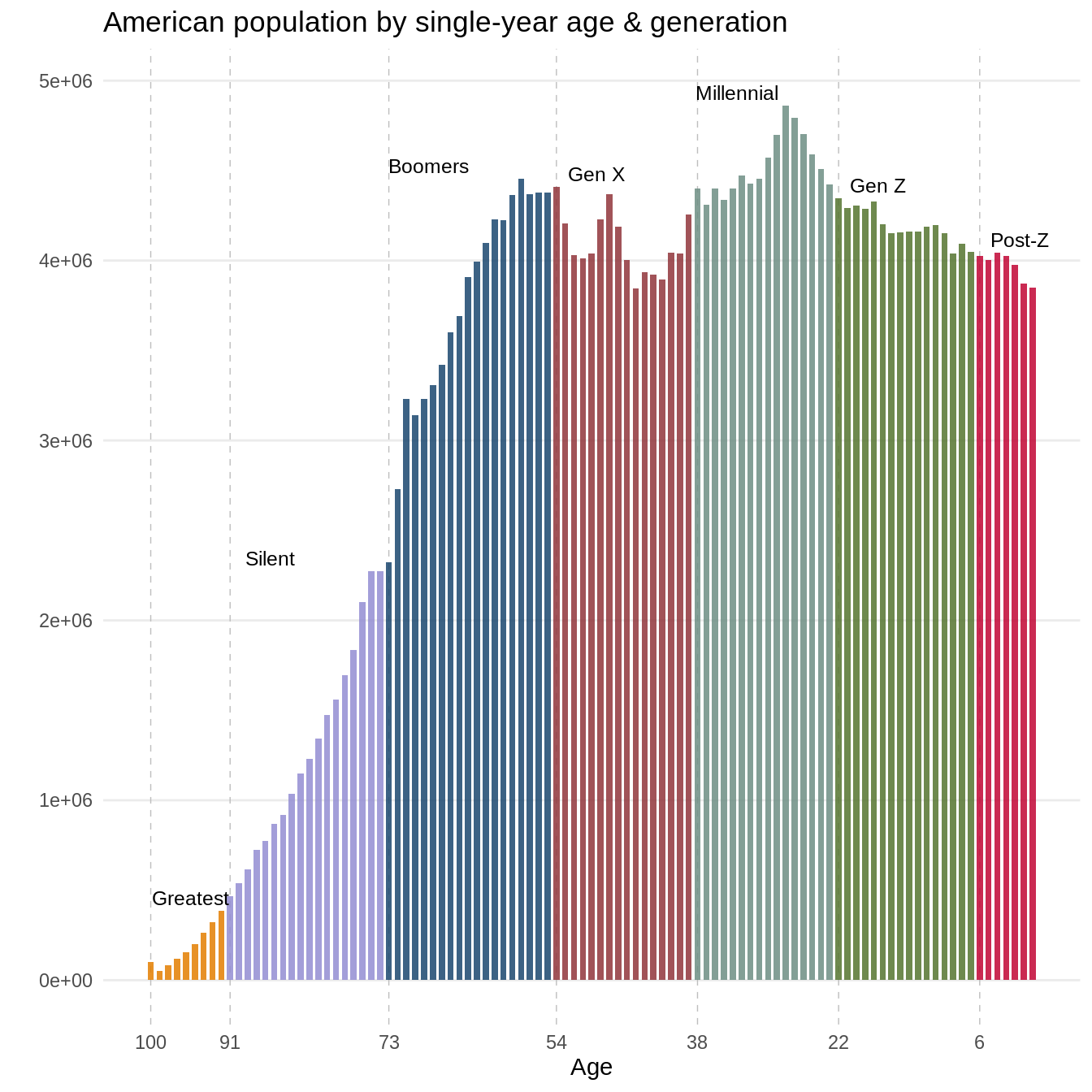### Population by single year of age, race & generation

gen_pal <- c('#b0bcc1','#ead8c3', '#437193',
'#c66767', '#55752f', '#dae2ba',
'#7b9bb3')

Next, we crosscut the single year of age counts presented above by race & ethnicity.

gen_pops %>%
ggplot(aes(x = AGE,
y = pop,
fill = race1)) +
geom_area(stat = "identity",
color = 'white',
alpha = 0.85) +
scale_fill_manual(values = gen_pal) +
geom_vline(xintercept = gg$AGE, linetype =2, color = 'gray', size = .25)+ annotate(geom="text", x = gg$AGE - 4.5,
y = gg$tot + 70000, label = gg$gen,
size = 3.25) +
xlab('')+ ylab('') +
theme_minimal() +
theme(legend.position="bottom",
legend.title = element_blank(),
panel.grid.major.x=element_blank(),
panel.grid.minor.x=element_blank(),
panel.grid.minor.y=element_blank()) +

scale_x_reverse(breaks = rev(gg$AGE) )+ labs(title ='American population by age, race & generation')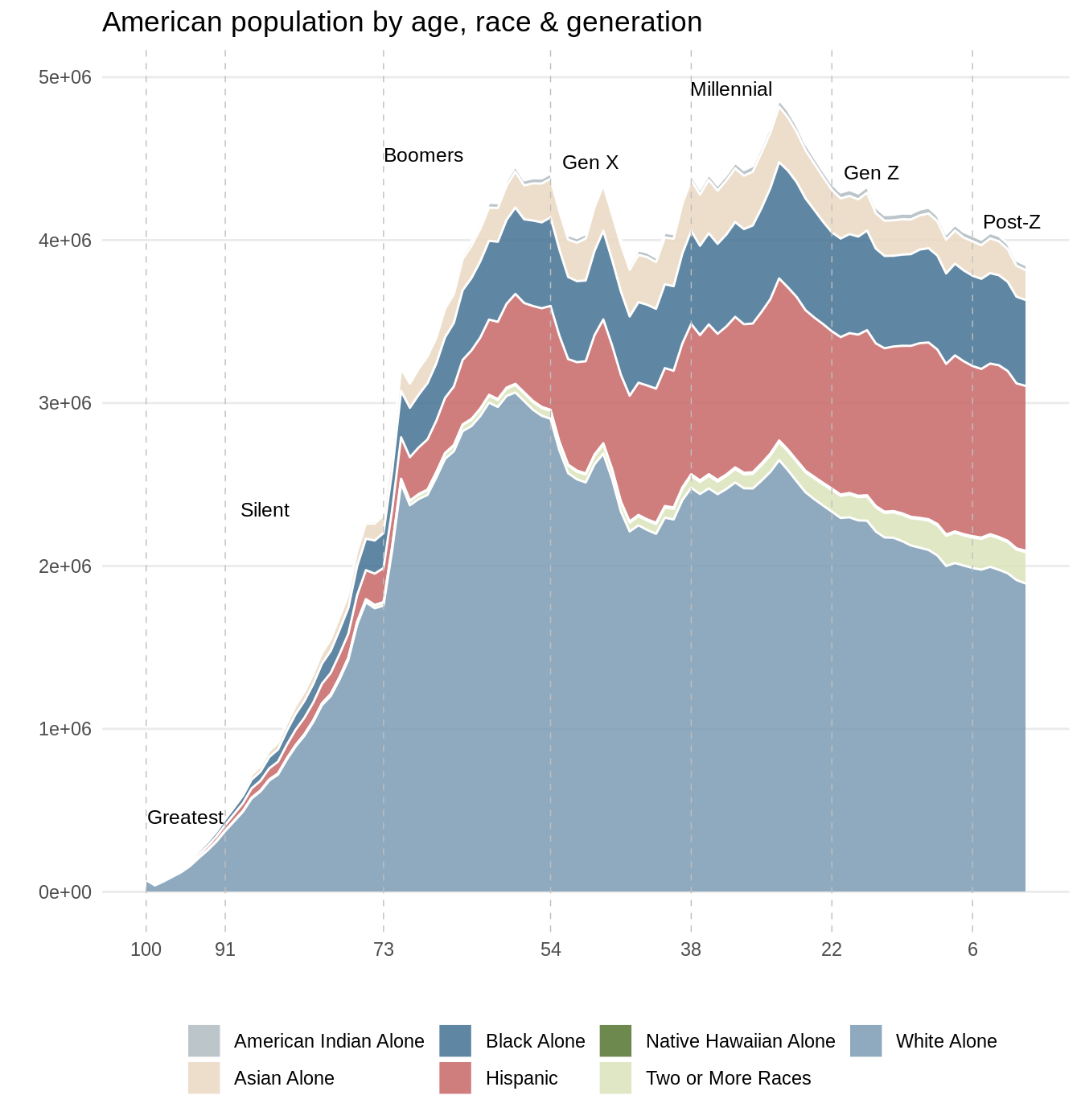### White America on the wane white_label <- gen_pops %>% group_by(gen, AGE) %>% mutate(per = pop/sum(pop))%>% filter(race1 == 'White Alone') %>% group_by(gen) %>% mutate(per = max(per)) %>% #For labels below. arrange(yob) %>% filter(yob %in% c('1919', '1928', '1946', '1965', '1981', '1997', '2013')) The last figure illustrates a proportional perspective of race & ethnicity in America by single year of age. Per figure, generational differences (at a single point in time) can shed light on (the direction of) potential changes in the overall composition of a given populace. As well as a view of what that populace may have looked like in the past. gen_pops %>% group_by(gen, AGE) %>% mutate(per = pop/sum(pop)) %>% ggplot(aes(x = (AGE), y = per, fill = race1)) + geom_area(stat = "identity", color = 'white', alpha = 0.85) + geom_hline(yintercept = .5, linetype = 4, color = 'white') + scale_fill_manual(values = gen_pal) + geom_vline(xintercept = gg$AGE,
linetype = 2,
color = 'gray',
size = .25)+
annotate(geom="text",
x = gg$AGE-4.5, y = white_label$per - .05,
label = gg$gen, size = 3.25) + xlab('')+ ylab('') + theme_minimal() + theme(legend.position="bottom", legend.title = element_blank(), panel.grid.major.x=element_blank(), panel.grid.minor.x=element_blank()) + scale_x_reverse(breaks = rev(gg$AGE)) +
labs(title = 'American population by age, race & generation')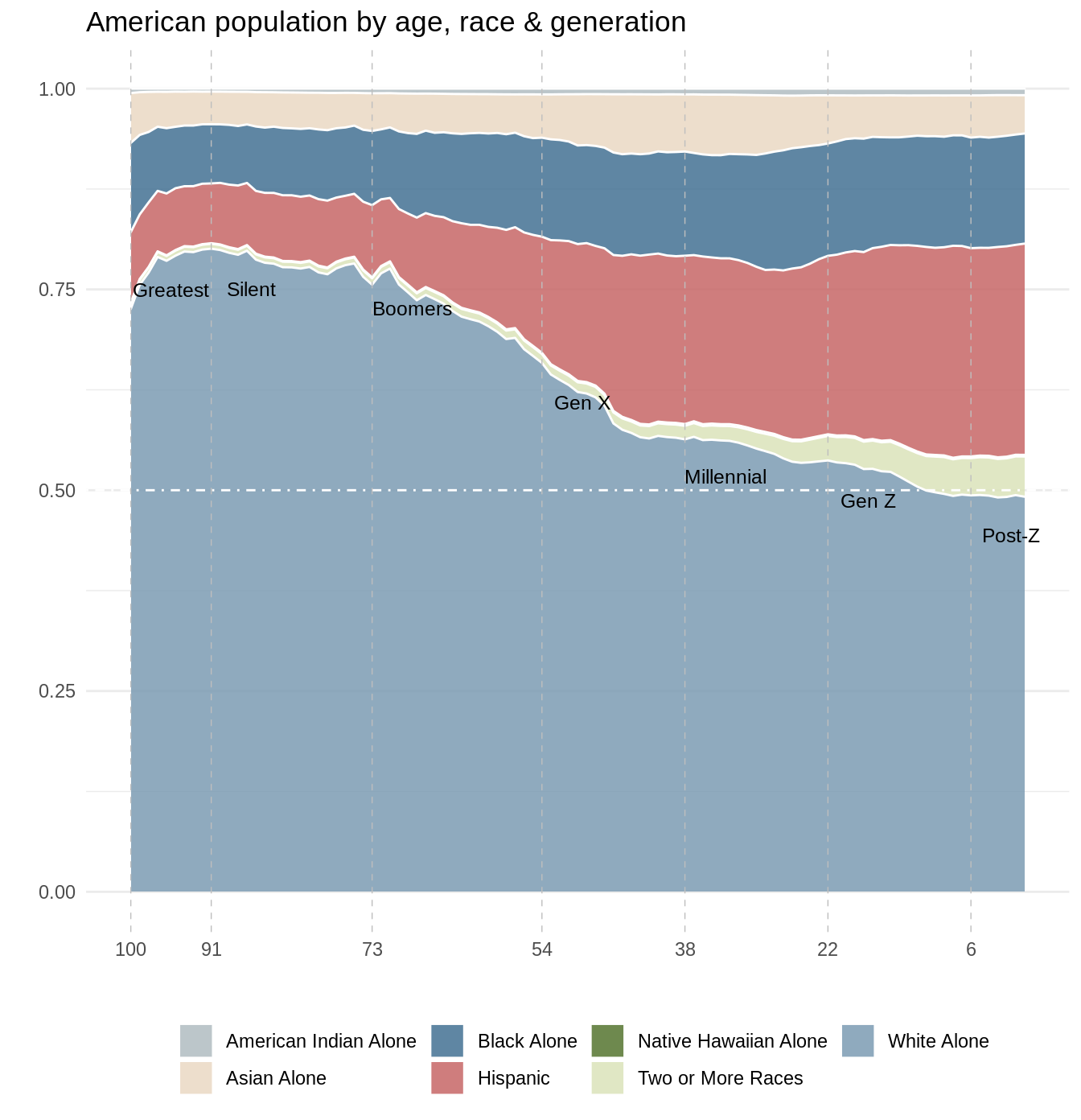## American generations in (apparent) time & space

Aggregate race-ethnicity profiles for America’s seven living generations are presented in the table below. Per American Community Survey (ACS) 2018 5-year estimates, the Gen X race-ethnicity profile (or distribution) is most representative of American demographics overall.

sums <- gen_pops %>%
group_by(rank, gen, race1) %>%
summarize(pop = sum(pop))%>%
group_by(gen) %>%
mutate(per = round(pop / sum(pop), 3))

### Race-ethnicity profiles for US counties

Here, we compare race-ethnicity profiles for US counties to those of American generations. Using the tidycensus R package, we first obtain county-level race-ethnicity estimates (ACS 2018 5-year);

#x <- tidycensus::load_variables(year = 2018, dataset = 'acs5/profile')
variable <- c('DP05_0071P', 'DP05_0077P', 'DP05_0078P',
'DP05_0079P', 'DP05_0080P',
'DP05_0081P', 'DP05_0082P',
'DP05_0083P')

variable_name <- c('Hispanic', 'White Alone', 'Black Alone',
'American Indian Alone', 'Asian Alone',
'Native Hawaiian Alone', 'Some Other Race Alone',
'Two or More Races')

gen <-  tidycensus::get_acs(geography = 'county',
variables = variable,
year = 2018,
survey = 'acs5') %>%
left_join(data.frame(variable, variable_name, stringsAsFactors = FALSE))%>%
select(-variable, -moe) %>%
rename(variable = variable_name) %>%
select(GEOID:NAME, variable, estimate)

then, via the tigris library, we obtain shapefiles for (1) all US counties and (2) US states.

nonx <- c('78', '69', '66', '72', '60', '15', '02')

library(tigris)
options(tigris_use_cache = TRUE,
tigris_class = "sf")

us_counties <- tigris::counties(cb = TRUE) %>%
filter(!STATEFP %in% nonx)
us_states <- tigris::states(cb = TRUE) %>%
filter(!STATEFP %in% nonx)

laea <- sf::st_crs("+proj=laea +lat_0=30 +lon_0=-95")
us_counties <- sf::st_transform(us_counties, laea)
us_states <- sf::st_transform(us_states, laea)

### Comparing distributions

The table below highlights the race-ethnicity distribution for my hometown, Dutchess County, NY. GEOID = 36027.

which1 <- gen %>%
mutate(estimate = ifelse(estimate == 0, .0000001, estimate)) %>%
inner_join(sums, by = c('variable' = 'race1')) %>%

mutate(div = estimate/100 * log((estimate/100)/per)) %>%
group_by(GEOID, NAME, gen) %>%
summarize (relative_entropy = round(sum(div), 4)) %>%
ungroup()

Via Kullback–Leibler divergence (ie, relative entropy), we compare the demographic profile of Dutchess County to the demographic profiles of each American generation. Per the table below, the Dutchess County profile is most similar to that of the Boomer generation. Per the lowest relative entropy value. This is not to say that there are more Boomers in Dutchess County; instead, the racial demographics of Dutchess County are most akin to an America when Boomers were in their prime.

which1 %>%
filter(GEOID == 36027) %>%
left_join(gen_desc) %>%
select(rank, NAME, gen, relative_entropy) %>%
arrange(rank) %>%
DT::datatable(rownames = FALSE,
options = list(dom = 't',
scrollX = TRUE,

rowCallback = DT::JS('function(row, data, index, rowId) {',
'console.log(rowId)','if(rowId == 2 ) {',
'row.style.backgroundColor = "#dae2ba";','}','}'))) 

which2 <- which1 %>%
group_by(GEOID, NAME) %>%
filter(relative_entropy == min(relative_entropy)) %>%
ungroup() 

So, while Gen X is most representative of America in the aggregate, per the map below, the demographics of America’s youngest & eldest generations are most prevalent at the county-level. Post-Z in the West, and the Greatest in the East & Midwest. (With Boomer & Millenial demographics most prevalent in the South.) New & Old Americas, perhaps.

us_counties %>%
left_join(which2, by = 'GEOID') %>%
ggplot() +
geom_sf(aes(fill = gen),  lwd = 0) +
ggthemes::scale_fill_stata() +
geom_sf(data=us_states,
fill = NA,
show.legend = F,
color="darkgray",
lwd=.5) +
theme(axis.title.x=element_blank(),
axis.text.x=element_blank(),
axis.title.y=element_blank(),
axis.text.y=element_blank(),
legend.position = 'bottom') +
labs(title = "Counties & Generations in America")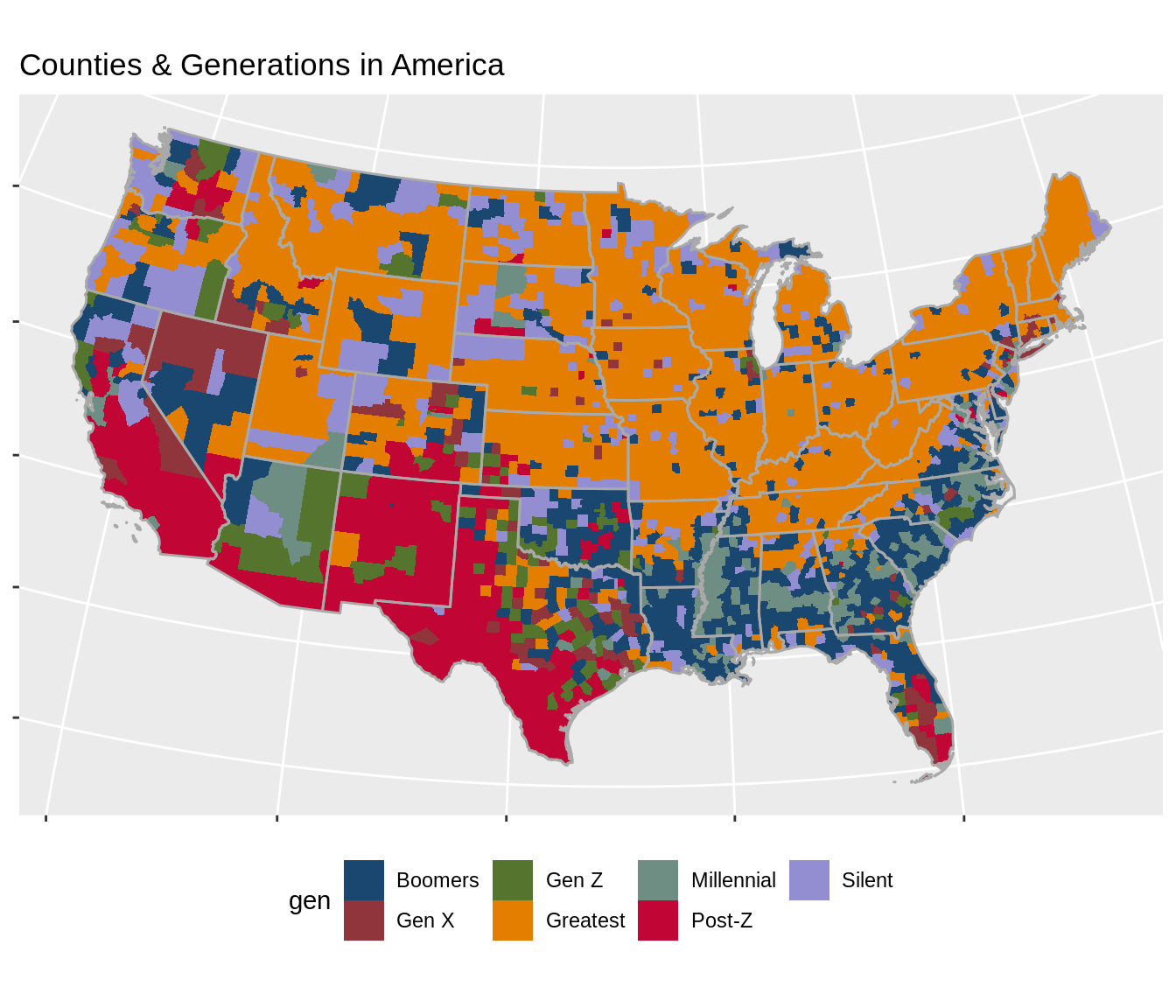## Summary

Some different perspectives on the composition of America & American generations, as well as a novel (static) take on the changing demographics of America.

Share